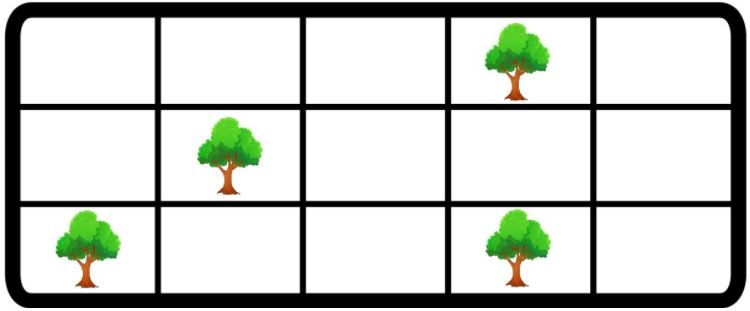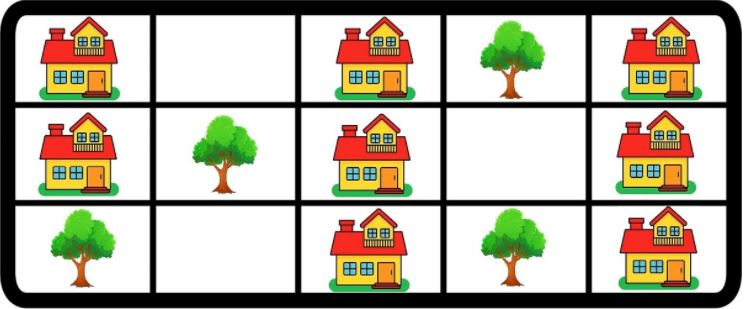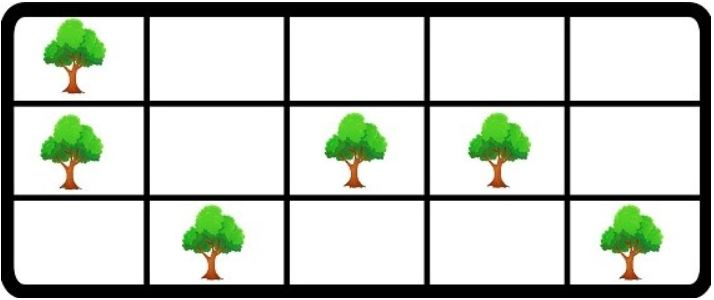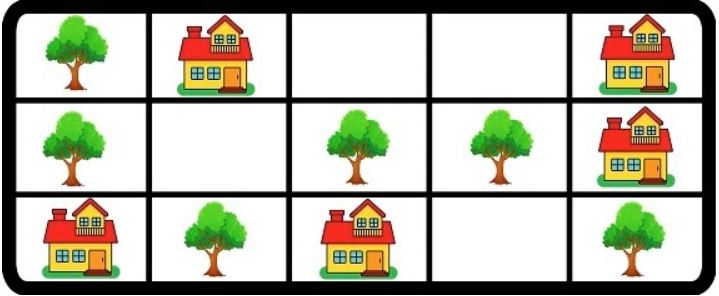0

# Town Planning

Difficulty: HARDAvg. time to solve
35 min
Success Rate
50%

Problem Statement

#### Ninja wants to meet the demands of the citizens without cutting any of the trees.

##### Following are the conditions asked by citizens for their house locations :
``````House should not have a house on its left cell
House should not have a house on its right cell
House should not have a house on its upper-left cell
House should not have a house on its upper-right cell
``````

#### For Example :

``````Input  :
M = 3 , N = 5
Grid :
. . . T .
. T . . .
T . . T .

In the example above, the maximum number of houses that be built is 8.
``````
##### Input Format :
``````The first line of input contains an integer ‘T’ denoting the number of test cases to run. Then ‘T’ test cases follow:

The first line in each test case contains two space-separated positive integers ‘M’ and ‘N’, where 'M’ is the number of rows and ‘N’ is the number of columns in the grid.

The next ‘M’ lines contain a string of length ‘N’ containing ‘.’ or ‘T’ representing empty space or a Tree, respectively.
``````
##### Output Format :
``````For each test case, print an integer denoting the maximum number of houses that can build following the conditions stated above.

Output for every test case will be printed in a separate line.
``````
##### Note :
``````You don’t need to print anything; It has already been taken care of. Just implement the given function.
``````
##### Constraints :
``````1 <= T <= 5
1 <= N <= 8
1 <= M <= 8
‘TOWN’ = { “.”,"T"}

Where 'TOWN’ denotes the 2-D grid represents whether a cell is empty or not.

Time limit: 1 sec
``````
##### Sample Input 1 :
``````1
3 5
. . . T .
. T . . .
T . . T .
``````
##### Sample Output 1 :
``````8
``````
##### Explanation of sample input 1 :
``````The following diagram represents the above input grid :
````````````The possible optimal arrangement of houses is shown below:
````````````Hence, a maximum 8 number of houses can be built if demands of citizens are to met and no tree is removed.
``````
##### Sample Input 2 :
``````1
3 5
T . . . .
T . T T .
. T . . T
``````
##### Sample Output 2 :
``````5
``````
##### Explanation of sample input 2 :
``````The following diagram represents the above input grid :
````````````The possible optimal arrangement of houses is shown below:
````````````Hence, a maximum 5 number of houses can be built if the demands of citizens are met and no tree is removed.
``````Console